# eSpark Learning: Factor Pairs Framing Video (4.OA Quest 3)

##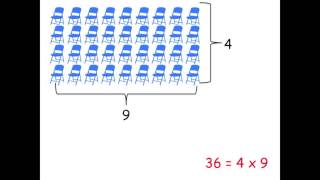By esparklearning videos

This eSpark video outlines a new learning quest that will help students master Common Core State Standard 4.OA: Find all factor pairs for a whole number between 1-100.# [3.OA.4-2.0] Unknowns in Mult/Div - Common Core Standard

##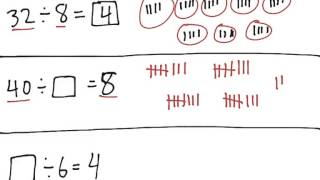By Freckle education

Determine the unknown whole number in a division equation relating three whole numbers. For example, determine the unknown number that makes the equation true in each of the equations 8 × ? = 48, 5 = _ ÷ 3, 6 × 6 = ?# [4.OA.1-1.0] Multiplication as Comparison - Common Core Standard

##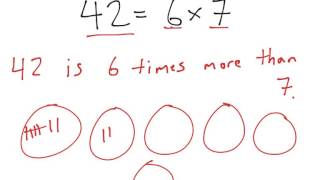By Freckle education

Interpret a multiplication equation as a comparison# [4.OA.4-2.0] Factor Pairs less than 100 - Common Core Standard

##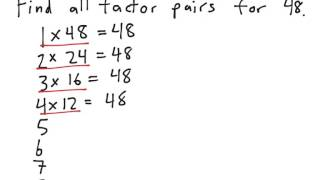By Freckle education

Find all factor pairs for a whole number in the range 1–100.# Finding Unknown Factors in Multiplication Equations: 3.OA.4

##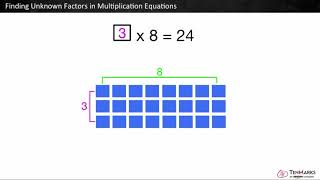By Tenmarks Amazon

Students learn to find unknown factors in multiplication equations using arrays and repeated addition.# Identifying and Creating Number Patterns (4.OA.5)

##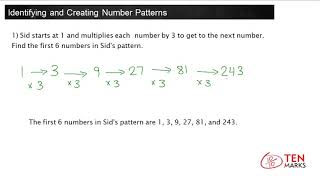By TenMarks Amazon

how to generate a pattern given a rule. It also shows how to identify a rule by completing a pattern given a sequence of numbers# [4.OA.3-1.0] Multi-step Word Problems - Common Core Standard

##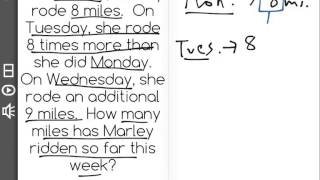By Freckle education

Solve multistep word problems posed with whole numbers and having whole-number answers using the four operations# 4.OA.3- Understanding Multi-step Problems(Grade 4 Math) |

##By Lumos Learning

This lesson was cerated to be ued in a 4th grade flipped Math class. (Slides provided by LearnZillion# [4.OA.5-1.0] Number/ Shape Patterns - Common Core Standard

##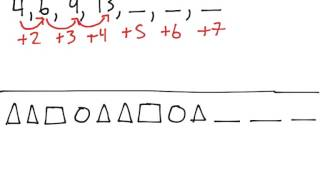By Freckle education

Generate a number or shape pattern that follows a given rule.# [4.OA.5-2.0] Number/ Shape Patterns - Common Core Standard

##By Freckle education

Identify apparent features of the pattern that were not explicit in the rule itself# 4th Grade Spatial Reasoning with Polygons

##By

4th grade math lesson on spatial reasoning with polygons, aligned to MA Curriculum Framework Standard 4.OA.5.# 4.OA.3- Understanding Multi-step Problems(Grade 4 Math) | www.lumoslearning.com

##By Lumos Learning

Using the Lumos Study Programs, parents and educators can reinforce the classroom learning experience for children and help them succeed at school and on the standardized tests. Lumos books, dvd, eLearning and tutoring are used by leading schools, libraries and thousands of parents to supplement classroom learning and improve student achievement in the standardized tests.##By Lumos Learning

Grade 4 lesson demonstrating CCSS Math Standard 4.OA..A.1,2, using literacy strategies to understand word problems. Lesson progresses through prior understandings of multiplication as groups of objects to multiplicative comparisons.##By

Grade 4 lesson demonstrating CCSS Math Standard 4.OA..A.1,2, using literacy strategies to understand word problems. Lesson progresses through prior understandings of multiplication as groups of objects to multiplicative comparisons.# [3.OA.4-2.0] Unknowns in Mult/Div - Common Core Standard

##By Front Row

Discover more Common Core Math at https://www.frontrowed.com Determine the unknown whole number in a division equation relating three whole numbers. For example, determine the unknown number that makes the equation true in each of the equations 8 Ãƒâ€” ? = 48, 5 = _ ÃƒÂ· 3, 6 Ãƒâ€” 6 = ?Front Row is a free, adaptive, Common Core aligned math program for teachers and students in kindergarten through eighth grade. Front Row allows students to practice math at their own pace - learning advanced concepts when they 're ready and receiving remediation when they struggle. Front Row provides teachers with access to a detailed data dashboard and weekly email reports that show which standards are causing students difficulty, what small groups can be formed for interventions, and how their students are progressing in math.Discover more Common Core Math at https://www.frontrowed.com# [4.OA.2-2.0] Word Problems: Mult Comparison - Common Core Standard

##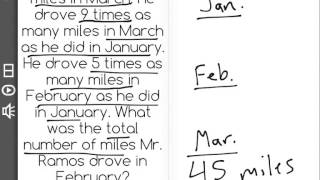By Freckle Education

Discover more Common Core Math at https://www.frontrowed.comDivide to solve word problems involving multiplicative comparison, e.g., by using drawings and equations with a symbol for the unknown number to represent the problem, distinguishing multiplicative comparison from additive comparison.1Front Row is a free, adaptive, Common Core aligned math program for teachers and students in kindergarten through eighth grade. Front Row allows students to practice math at their own pace - learning advanced concepts when they 're ready and receiving remediation when they struggle. Front Row provides teachers with access to a detailed data dashboard and weekly email reports that show which standards are causing students difficulty, what small groups can be formed for interventions, and how their students are progressing in math.Discover more Common Core Math at https://www.frontrowed.com# eSpark Learning: Prime and Composite Numbers Framing Video (4.OA Quest 4)

##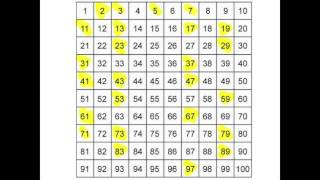By eSparkLearningVideos# 4.OA.2-Understanding of real word problems(Grade 4 Math) | www.lumoslearning.com

##By Lumos Learning

Using the Lumos Study Programs, parents and educators can reinforce the classroom learning experience for children and help them succeed at school and on the standardized tests. Lumos books, dvd, eLearning and tutoring are used by leading schools, libraries and thousands of parents to supplement classroom learning and improve student achievement in the standardized tests.# 2.2 comparison problems

##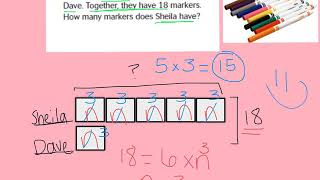By Kjuarezmath

world problems comparison# Finding the Missing Factor

##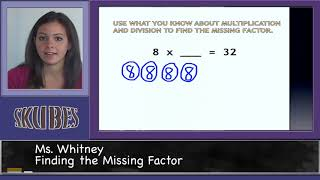By Skubes ed

Determine the unknown whole number in a multiplication or division equation relating three whole numbers. For example, determine the unknown number that makes the equation true in each of the equations 8 x ? = 48, 5 = _ ÷ 3, 6 x 6 = ?